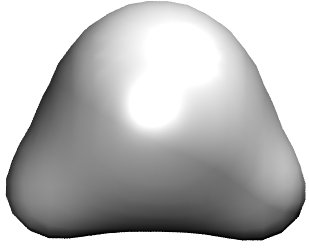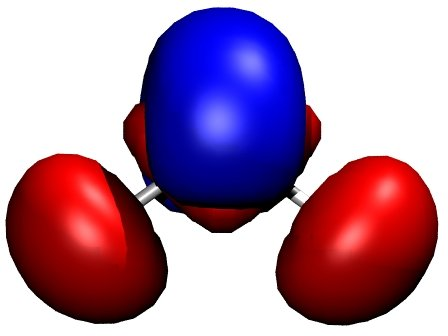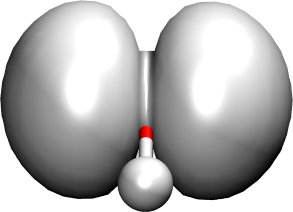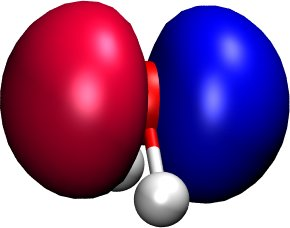First steps with Waveplot¶

[Input: recipes/basics/waveplot/]

Waveplot is a tool for generating grid-based volumetric data for charge distributions or wave-functions in your system. By visualising those distributions with appropriate graphical tools you can obtain a deeper understanding of the physics and chemistry of your quantum mechanical system.

Making a DFTB+ calculation¶

In order to plot the charge distribution or the orbitals in a certain system, you have to execute a DFTB+ calculation for this system first. The calculation must be executed as usual, you just have to make sure, that the options WriteDetailedXML and WriteEigenvectors are turned on.

Below you see the input for the H2O molecule, where the geometry is optimised by DFTB+:

Geometry = GenFormat {
3  C
O H
1    1    0.00000000000E+00  -0.10000000000E+01   0.00000000000E+00
2    2    0.00000000000E+00   0.00000000000E+00   0.78306400000E+00
3    2    0.00000000000E+00   0.00000000000E+00  -0.78306400000E+00
}

MovedAtoms = 1:-1
MaxForceComponent = 1.0e-4
MaxSteps = 100
OutputPrefix = "geom.out"
}

Hamiltonian = DFTB {
Scc = Yes
SccTolerance = 1.0e-5
SlaterKosterFiles = Type2FileNames {
Prefix = "../../slakos/mio-ext/"
Separator = "-"
Suffix = ".skf"
}
MaxAngularMomentum = {
O = "p"
H = "s"
}
}

Options {
WriteDetailedXml = Yes
WriteEigenvectors = Yes
}

ParserOptions {
ParserVersion = 7
}

Running DFTB+ for this input, you should obtain the usual results, and additionaly the files detailed.xml and eigenvec.bin. Former contains some information about the calculated system, latter contains the obtained eigenvectors in binary format. Both files are needed by waveplot.

Running Waveplot¶

Now, you have to decide, what kind of charge distributions, wavefunctions etc. to plot. In the current example, we will plot the total charge distribution of the water molecule, the charge distribution (wavefunction squared) for the highest occupied molecular orbital (HOMO), the wave function for the HOMO, and the total charge difference, which tells us, how the chemical bonding between the atoms modified the total charge distribution compared to the superpositions of neutral atomic densities.

Input¶

The appropriate waveplot input (waveplot_in.hsd) could look like the following:

# General options

Options {
TotalChargeDensity = Yes           # Total density be plotted?
TotalChargeDifference = Yes        # Total density difference plotted?
ChargeDensity = Yes                # Charge density for each state?
RealComponent = Yes                # Plot real component of the wavefunction
PlottedSpins = 1 -1
PlottedLevels = 4                  # Levels to plot
PlottedRegion =  OptimalCuboid {}  # Region to plot

NrOfPoints = 50 50 50              # Number of grid points in each direction
NrOfCachedGrids = -1               # Nr of cached grids (speeds up things)
Verbose = Yes                      # Wanna see a lot of messages?
}

DetailedXml = "detailed.xml"         # File containing the detailed xml output
# of DFTB+
EigenvecBin = "eigenvec.bin"         # File cointaining the binary eigenvecs

# Definition of the basis
Basis {
Resolution = 0.01
# Including mio-1-1.hsd. (If you use a set, which depends on other sets,
# the wfc.*.hsd files for each required set must be included in a similar
# way.)
<<+ "../../slakos/wfc/wfc.mio-1-1.hsd"
}

Some notes to the input:

• Option TotalChargeDensity controls the plotting of the total charge density. If turned on, the file wp-abs2.cube is created.

• Option TotalChargeDifference instructs Waveplot to plot the difference between the actual total charge density and the density you would obtain by summing up the densities of the neutral atoms.

• Option ChargeDensity tells the code, that the charge distribution for some orbitals (specified later) should be plotted. Similarly, RealComponent instructs Waveplot to create cube files for the real part of the one-electron wavefunctions for the specified orbitals. (For non-periodic systems the wavefunctions are real.)

• Options PlottedSpins, PlottedLevels (for periodic systems also PlottedKPoints) controls the levels (orbitals) to plot. In the current example we are plotting level 4 (is the HOMO of the water molecule) for all available spins. Since the DFTB+ calculation was spin unpolarised, we obtain only one plot for the HOMO in file wp-1-1-4-abs2.cube (1-1-4 in the file name indicates first K-point, first spin, 4th level).

• The region to plot is selected with the option PlottedRegion. Instead of specifying the box origin and box dimensions by hand, Waveplot can be instructed by using the OptimalCuboid method to take the smallest cuboid, which contains all the atoms and enough space around them, so that the wavefunctions are not leaking out of it. (For details and other options for PlottedRegion please consult the manual.) The selected region in the example is sampled by a mesh of 50 by 50 by 50. (NrOfPoints)

• The basis defintion (Basis) is made by including the file containing the appropriate wave function coefficient definitions. You must make sure that you use the file for the same set, which you used during your DFTB+ calculation. Here, the mio-1-1 set was used for calculating the H2O molecule, and therefore the file wfc.mio-1-1.hsd is included.

The wavefuntion coefficients can be usually downloaded from the same place as the Slater-Koster files.

Output¶

================================================================================
WAVEPLOT  0.2
================================================================================

Interpreting input file 'waveplot_in.hsd'
--------------------------------------------------------------------------------
WARNING!
-> The following 3 node(s) had been ignored by the parser:
(1)
Path: waveplot/Basis/C
Line: 1-33 (File: wfc.mio-0-1.hsd)
(2)
Path: waveplot/Basis/N
Line: 52-84 (File: wfc.mio-0-1.hsd)
(3)
Path: waveplot/Basis/S
Line: 120-170 (File: wfc.mio-0-1.hsd)

Processed input written as HSD to 'waveplot_pin.hsd'
Processed input written as XML to 'waveplot_pin.xml'
--------------------------------------------------------------------------------

Doing initialisation

Starting main program

Origin
-5.00000 -6.35306 -6.47114
Box
10.00000 0.00000 0.00000
0.00000 11.08472 0.00000
0.00000 0.00000 12.94228
Spatial resolution [1/Bohr]:
5.00000 4.51071 3.86331

Total charge of atomic densities:    7.981973

Spin KPoint  State  Action        Norm   W. Occup.

Calculating grid

1      1      1    calc    0.996855    2.000000
1      1      2    calc    1.003895    2.000000
1      1      3    calc    0.998346    2.000000
1      1      4    calc    1.000053    2.000000
File 'wp-1-1-4-abs2.cube' written
File 'wp-1-1-4-real.cube' written
File 'wp-abs2.cube' written

Total charge:    7.998297

File 'wp-abs2diff.cube' written

================================================================================

Some notes on the output:

• The warnings about unprocessed nodes appears, because the included file wfc.mio-0-1.hsd also contained wave function coefficients for elements (C, N, S), which are not present in the calculated system. Hence these extra definitions in the file were ignored.

• The Total charge of atomic densities tells you the amount of charge found in the selected region, if atomic densities are superposed. This number should be approximately equal to the number of electrons in your system (here 8). There could be two reasons for a substantial deviation. Either the grid is not dense enough (option NrOfPoints) or the box for the plotted region is too small or misplaced (PlottedRegion).

• The output files for the individual levels (charge density, real part, imaginary part) follow the naming convention wp-KPOINT-SPIN-LEVEL-TYPE.cube.

The total charge and the total charge difference are stored in the files wp-abs2.cube and wp-abs2diff.cube, respectively.

Visualising the results¶

The volumetric data generated by Waveplot is in the Gaussian cube format and can be visualized with several graphical tools (VMD, JMol, ParaView, …). Below we show the necessary steps to visualize it using VMD. (It refers to VMD version 1.8.6 and may differ in newer versions.)

Total charge distribution¶

The cube file containing the total charge distribution wp-abs2.cube can be read by using the File|New Molecule menu. VMD should automatically recognise, that the file has the Gaussian cube format. After successful loading, the VMD screen shows the skeleton of the molecule.

In order to visualise the charge distribution, the graphical representation of the molecule has to be changed. This can be achieved by using the Graphics|Representations... submenu. The skeleton representation can be turned to a CPK represenation (using balls and sticks) by selecting CPK for the Drawing method in the Graphical Representations dialog box. Then you should create an additional representation (Create Rep) and change the drawing method for it to be Isosurface. The type of isosurface (Draw) should be changed from Points to Solid Surface and instead of Box+Isosurface only Isosurface should be selected. Then, by tuning the Isovalue one can select the isosurface to be plotted. Figure 1 was created using 0.100. (Display background color had been set to white using the Graphics|Colors menu.)Figure 1 Total charge density for the H2O molecule, created by Waveplot, visualised by VMD.

Charge distribution difference¶

The charge distribution difference can be plotted in a similar way as the total charge. One has to load the file wp-abs2diff.cube. One should then, however, make not one, but two additional graphical representations of the type Isosurface. One of them should have positive isovalue, the other one a negative one. The different isosurfaces can be colored in a different way by using ColorID as coloring method and choosing different color values for the different representations.

Figure 2 demonstrates this for the water molecule. Negative net populations were colored red, positive net populations blue. One can clearly see, that there is a significant electron transfer from the hydrogens to the oxygen (lone pair on the oxygen).Figure 2 Charge density difference (total density minus sum of atomic densities) for the H2O molecule, as created by Waveplot and visualised by VMD.

Molecular orbitals¶

The plotting of molecular orbitals can be, depending which property is plotted, done in the same way as the total charge distribution or the total charge difference. If the charge density (probability distribution) of an orbital is plotted, the data contains only positive values, therefore only one isosurface representation is necessary (like for the charge distribution). If the real (or for periodic systems also the imaginary) part of the wavefunction is to be plotted, two isosurface representations are needed, one for the positive and one for the negative values (like for the charge difference).

Figure 3 shows the distribution of the electron (wavefunction squared) for the HOMO, while Figure 4 shows the HOMO wavefunction itself (blue - positive, red - negative). You can easily recognise the p-type of the HOMO, positive on one side, negative on the other side, a node plane in the middle.Figure 3 Highest occupied molecular orbital of a water molecule (wavefunction square)Figure 4 Highest occupied molecular orbital of a water molecule (real part of the wavefunction).